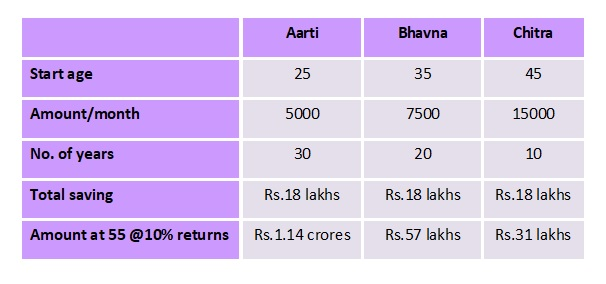# The Wealth Architects

(Precious) Words of Wisdom : "Never spend your money before you have earned it." ~ Thomas Jefferson

# How to calculate Compounded Annual Growth Rate (CAGR)

Let us begin this post with an example.

Suppose you had invested Rs.10,000 in a mutual fund scheme, four years back. Today, that investment is valued at Rs.20,000.

Your profit is equal to Rs.10,000.

So is your profit 100% (= Profit / Investment*100 = 10,000/10,000*100)?

No, that would be wrong:

Why? Simple, because the above formula (of simply dividing the profit by investment), does not account for the four years you were invested in the scheme.

It would be more appropriate to calculate profit per annum. This would enable you to make a more meaningful comparison with other investments such as the rate of interest on your fixed deposits or PPF, etc.

So is your profit 25% (=Profit % / no. of years  = 100% / 4).

Again, no! Simply dividing the total returns by the total number of years is wrong:

Why? Simple, because this simple formula does not account for the return on return every year i.e. the compounding effect.

Instead, you have to calculate what is commonly referred to as the Compounded Annual Growth Rate or the CAGR.

#### What is CAGR?

CAGR is the year-on-year growth rate of an investment, over a specified period of time. The formula for calculating the same is as under:

CAGR = (Final Value / Initial Investment)^(1 / No. of years) - 1
= (20000 / 10000)^(1 / 4) - 1
= 2^0.25 - 1
= 1.1892 - 1
= 0.1892

Hence, in the above example, the effective return works out to 18.92% p.a.

In other words, you earn 18.92% returns compounded annually.

So, after one year your earnings would be Rs.1,892 and total investment would be valued at Rs.11,892.

At the end of year 2: Earning is Rs.2250 (=18.92% of Rs.11,892) and Total Value is Rs.14,142.

Similarly,
At the end of year 3: Earning is Rs.2676 (=18.92% of Rs.14,142) and Total Value is Rs.16,818.
and
At the end of year 4: Earning is Rs.3182 (=18.92% of Rs.16,818) and Total Value is Rs.20,000.

Beware!

It, however, does not mean that EVERY year you earn the same returns. CAGR merely calculates the AVERAGE annualized return on your investment

Thus, it is very useful in calculating the average returns from an investment where the returns are market-linked and varying year-on-year e.g. equity, equity-based mutual funds, gold, etc.

Also, as mentioned earlier, it is also a more meaningful figure to use when comparing returns offered by different products.

## An Investment In Knowledge Pays The Best Interest ~ Benjamin Franklin## Ignorance is like a SIGNED BLANK CHEQUE... anyone can MISUSE it.

Subscribe via Email

## ... Three VALUABLE Tips ...

 1. Which Is The Best Day To Invest Your Money?My "unique" take on the auspicious days to make the best investments. 2. Housewives Too Can Become MillionairesSaving for MORE TIME gives much better results than saving MORE MONEY. 3. Twist In Credit Card Interest Calculations You Must KnowCalculating Finance Charge on your Credit Card is LOT SIMPLER than this.